Courses

# RD Sharma Solutions -Ex-23.2 (Part - 2), Graphical Representation Of Statistical Data, Class 9, Math Class 9 Notes | EduRev

## Class 9 : RD Sharma Solutions -Ex-23.2 (Part - 2), Graphical Representation Of Statistical Data, Class 9, Math Class 9 Notes | EduRev

The document RD Sharma Solutions -Ex-23.2 (Part - 2), Graphical Representation Of Statistical Data, Class 9, Math Class 9 Notes | EduRev is a part of the Class 9 Course RD Sharma Solutions for Class 9 Mathematics.
All you need of Class 9 at this link: Class 9

Q10: The following data gives the production of food grains (In thousand tonnes) for some years:

 Year 1995 1996 1997 1998 19999 2000 Production (in thousand rupees) 120 150 140 180 170 190

Represent the above data with the help of a bar graph.

Ans:

To represent the given data by a vertical bar graph, we first draw horizontal and vertical axes.

Let us consider that the horizontal and vertical axes represent the years and the production of food grains in thousand tonnes respectively. We have to draw 6 bars of different lengths given in the table. At first, we mark 6 points in the horizontal axis at equal distances and erect rectangles of the same width at these points. The heights of the rectangles are proportional to the production of food grains. The vertical bar graph of the given data is following: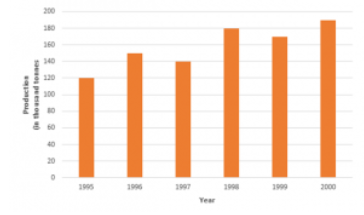Q11: The following data gives the amount of manure (in thousand tones) manufactured by a company during some years:

 Year 1992 1993 1994 1995 1996 1997 Manure(in thousand tones) 15 35 45 30 40 20

(i) Represent the above data with the help of a bar graph.

(ii) Indicate with the help of the bar graph the year in which the amount of manufactured by the company was maximum.

(iii) Choose the correct alternative:

The consecutive years during which there was the maximum decrease in manure production are:

(a) 1994 and 1995       (b) 1992 and 1993       (c) 1996 and 1997       (d) 1995 and 1996

Ans: To represent the given data by a vertical bar graph, we first draw horizontal and vertical axes. Let us consider that the horizontal and vertical axes represent the years and the amount of manure in thousand 9 ones respectively. We have to draw 6 bars of different lengths given in the table. At first, we mark 6 points in the horizontal axis at equal distances and erect rectangles of the same width at these points. The heights of the rectangles are proportional to the amount of manures manufactured by the company.

(1) The vertical bar graph of the given data is following: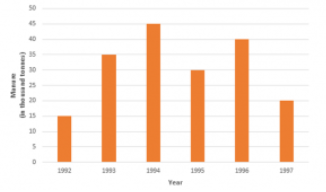(2) It is seen from the bar graph that the height of the 3s bar from the left is maximum, which is corresponding to the year 1994. So in 1994, the quantity manufactured by the company was maximum.

(3) It is seen from the bar graph that the manure production is decreased in the years 1995 (1.5 scale divisions) and 1997 (2 full-scale divisions). So, the maximum decrease is in the year 1997. Hence, the correct choice is (c).

Q12: The following data gives the demand estimates of the Government of India, Department of Electronics for the personnel in the Computer sector during the Eighth Plan period (1990-95):

 Qualifications MCA(masters in computer applications) DCA(diploma in computer applications DCE(diploma in computer engineering) CL(certificate level course) ST(short term course) Personnel required 40600 181600 18600 670600 1802900

Represent the data with the help of a bar graph. Indicate with the help of the bar graph the course where the estimated requirement is least.

Ans: To represent the given data by a vertical bar graph, we first draw horizontal and vertical axes. Let us consider that the horizontal and vertical axes represent the qualifications and the personnel required in hundreds respectively. We have to draw 5 bars of different lengths given in the table. At first, we mark 5 points in the horizontal axis at equal distances and erect rectangles of the same width at these points. The heights of the rectangles are proportional to the number of personnel required.

The vertical bar graph of the given data is following: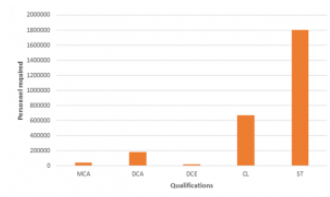It is seen from the bar graph that the height of the 3ffl bar from the left is least, which is corresponding to DCE. Hence, the requirement is least in DCE.

Q13: The income and expenditure for 5 years of a family is given in the following data:

Represent the above data by a bar graph.

 Years 1995-96 1996-97 1997-98 1998-99 1999-2000 Income (Rs. In thousands) 100 140 150 170 210 Expenditure (Rs. in thousands) 80 130 145 160 190

Ans: To represent the given data by a vertical bar graph, we first draw horizontal and vertical axes. Let us consider that the horizontal and vertical axes represent the years and the income or expenditure in thousand rupees respectively. We have to draw 5 bars for each income and expenditure side by side of different lengths given in the table.

At first, we mark 5 points for each income and expenditure in the horizontal axis at equal distances and erect rectangles of the same width at these points. The heights of the rectangles are proportional to the corresponding income or expenditures.

Q14: The investments (in ten crores of rupees) of Life Insurance Corporation of India in different sectors is given below:

 Sectors Investment (in ten crores of rupess) Central government securities 45 State government securities 11 Securities guaranteed by the government 23 Private sectors 18 Socially oriented sectors(plan) 46 Socially oriented sectors(Non – plan) 11

Represent the above data with the help of a bar graph.

Ans:

To represent the given data by a vertical bar graph, we first draw horizontal and vertical axes. Let us consider that the horizontal and vertical axes represent the sectors and the investment in ten Crores of rupees respectively. We have to draw 6 bars of different lengths given in the table. At first, we mark 6 points in the horizontal axis at equal distances and erect rectangles of the same width at these points. The heights of the rectangles are proportional to the investments of Life Insurance Corporation of India. The vertical bar graph of the given data is following: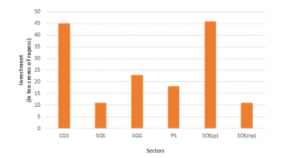The short forms used in the graph are:

(a) C.G.S. : Central Government Securities

(b) S.G.S. : State Government Securities

(c) S.G.G. : Securities Guaranteed by Government

(d) RS.: Private Sectors

(e) S.O.S.(P) : Socially Oriented Sectors (Plan)

(f) S.O.S.(NP) : Socially Oriented Sectors (Non-Plan)

Q15: The following data gives the value (in crores of rupees) of the Indian export of cotton textiles for different years:

 Years 1982-83 1983-84 1984-85 19985-86 19986-87 Value of exports of cotton textiles(in crores of rupees) 300 325 475 450 550

Represent the above data with the help of a bar graph. Indicate with the help of a bar graph the year in which the rate of increase in exports is maximum over the preceding year.

Ans: To represent the given data by a vertical bar graph, we first draw horizontal and vertical axes. Let us consider that the horizontal and vertical axes represent the years and the value of Indian export of cotton textiles in Crores of rupees respectively. We have to draw 5 bars of different lengths given in the table.

At first, we mark 5 points in the horizontal axis at equal distances and erect rectangles of the same width at these points. The heights of the rectangles are proportional to the values of Indian export of cotton textiles in different years. The vertical bar graph of the given data is following: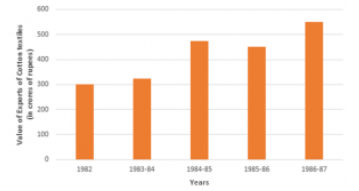The export increases in the years 1983-84, 1984-85 and 1986-87. Now,

(a) The rate of increase in the year 1983-84 is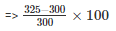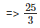= 8.33%

(b) The rate of increase in the year 1984-85 is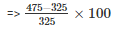=> 15000/ 325

=> 600/13

=> 46.15%

(c) The rate of increase in the year 1986-87 is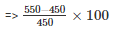=> 10000 /450

=> 22.22%

Hence, in the year 1984-85, the rate of increase of export is the maximum over the preceding year.

Q16: The following table gives the quantity of goods (in crores of rupees)

 Year 1950-51 1960-61 1965-66 1970-71 1980-81 1982-83 Quantity of goods(in crore tonnes) 9 16 20 20 22 26

Represent this information with the help of a bar graph.

Explain through the bar graph if the quantity of goods carried by the Indian Railways in 1965-66 is more than double the quantity of goods carried in the year 1950-51.

Ans: To represent the given data by a vertical bar graph, we first draw horizontal and vertical axes. Let us consider that the horizontal and vertical axes represent the years and the quantity of goods in Crores tonnes respectively. We have to draw 6 bars of different lengths given in the table. At first, we mark 6 points in the horizontal axis at equal distances and erect rectangles of the same width at these points. The heights of the rectangles are proportional to the quantity of goods carried by Indian railways in different years.

The vertical bar graph of the given data is following: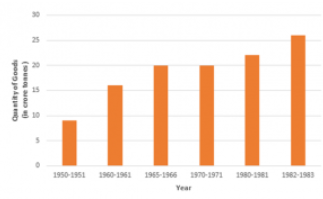It is seen from the bar graph that the quantity of goods carried in the years 1950-51 and 1965-66 are 20 Crores tonnes and 9 Crores tonnes. Clearly 20 is more than 2 multiplied by 9. Hence, the statement is true.

Q17: The production of oil (in lakh tonnes) in some of the refineries in India during 1982 was given below:

 Refinery Barauni Koyali Mathura Mumbai Florida Production of oil(in lakh tonnes) 30 70 40 45 25

Construct a bar graph to represent the above data so that the bars are drawn horizontally.

Ans: To represent the given data by a vertical bar graph, we first draw horizontal and vertical axes. Let u consider that the vertical and horizontal axes represent the refineries and the production of oil in la] tonnes respectively. We have to draw 5 bars of different lengths given in the table.

At first, we mark 5 points in the vertical axis at equal distances and erect rectangles of the same wk at these points. The lengths of the rectangles are proportional to the productions of oil.

The horizontal bar graph of the given data is following: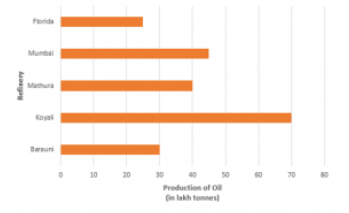Q18: The expenditure (in 10 crores of rupees) on health by the Government of India during the various five-year plans is shown below:

 Plans I II III IV V VI Expenditure on health(in 10 crores of rupees) 7 14 23 34 76 182

Construct a bar graph to represent the above data.

Ans: To represent the given data by a vertical bar graph, we first draw horizontal and vertical axes.

Let us consider that the horizontal and vertical axes represent the years and the expenditures on health in 10 Crores rupees respectively. We have to draw 6 bars of different lengths given in the table.

At first, we mark 6 points in the horizontal axis at equal distances and erect rectangles of the same width at these points. The heights of the rectangles are proportional to the expenditures on health by the government of India in different years.

The vertical bar graph of the given data is following: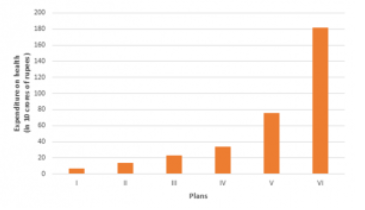Offer running on EduRev: Apply code STAYHOME200 to get INR 200 off on our premium plan EduRev Infinity!

91 docs

,

,

,

,

,

,

,

,

,

,

,

,

,

,

,

,

,

,

,

,

,

,

,

,

,

,

,

,

,

,

;